# 如何用Python和机器学习训练中文文本情感分类模型？

+关注继续查看# 疑惑# 数据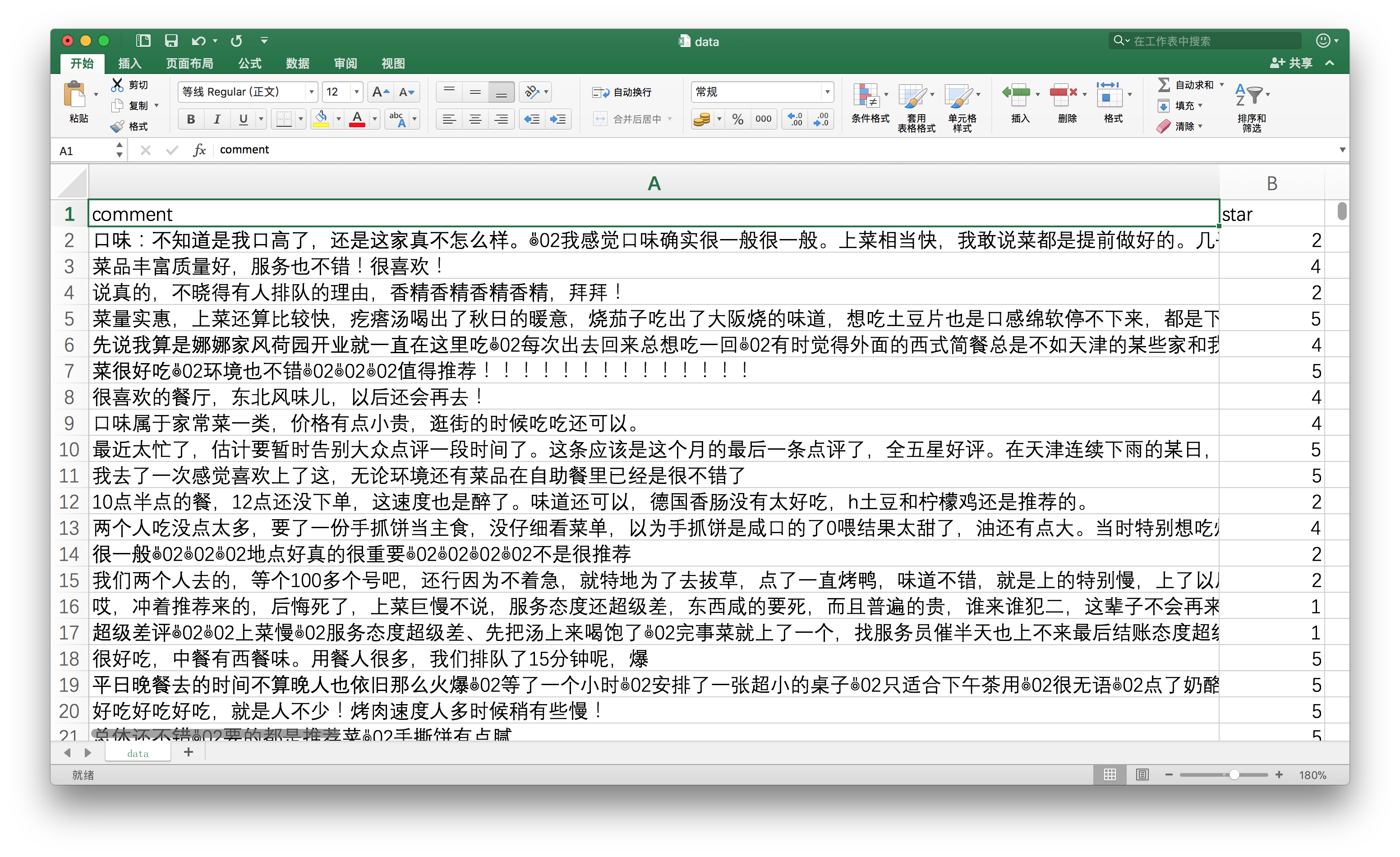# 模型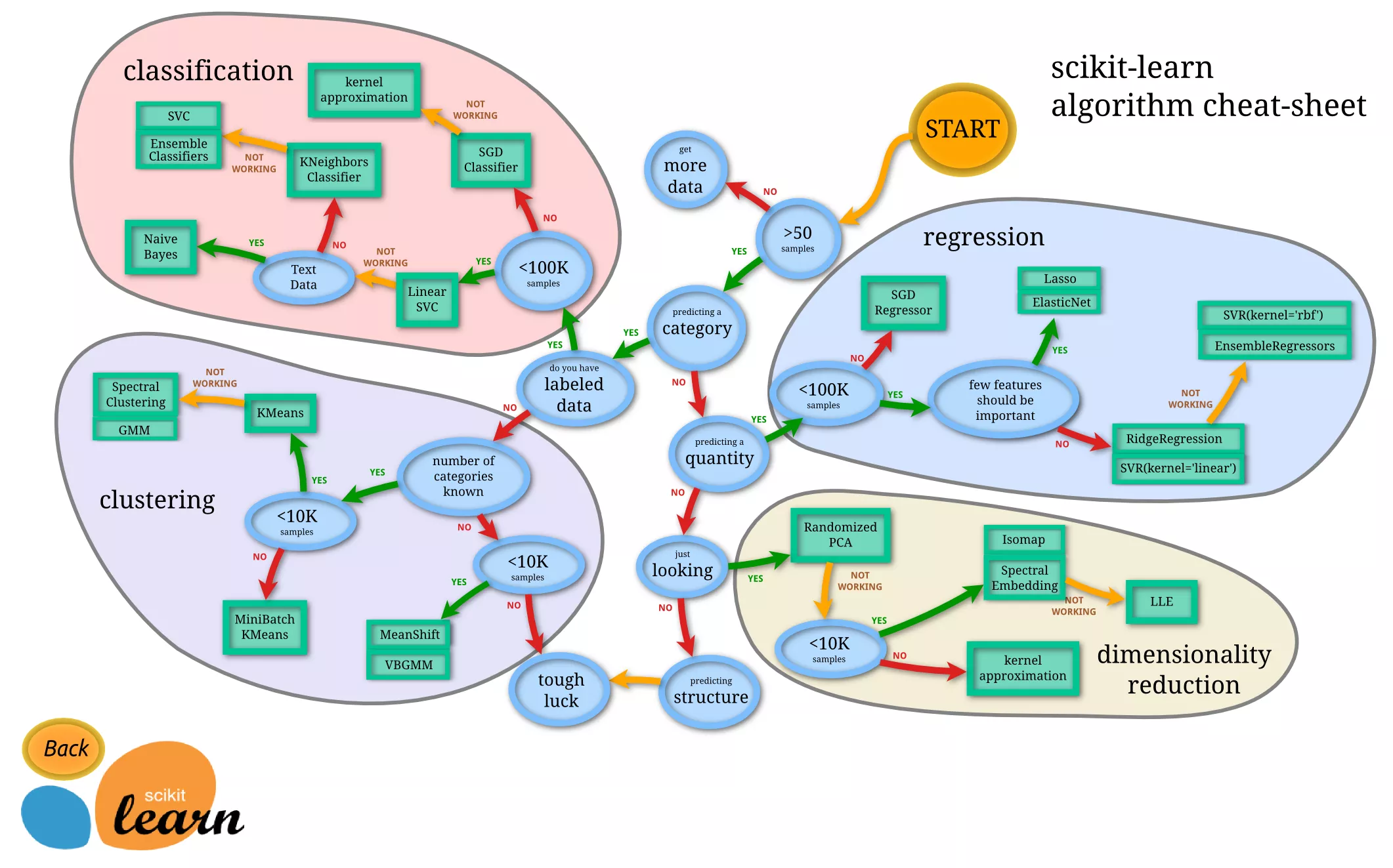Scikit-learn告诉我们：用朴素贝叶斯模型好了。

# 向量化

I love the game.

I hate the game.

• I
• love
• hate
• the
• game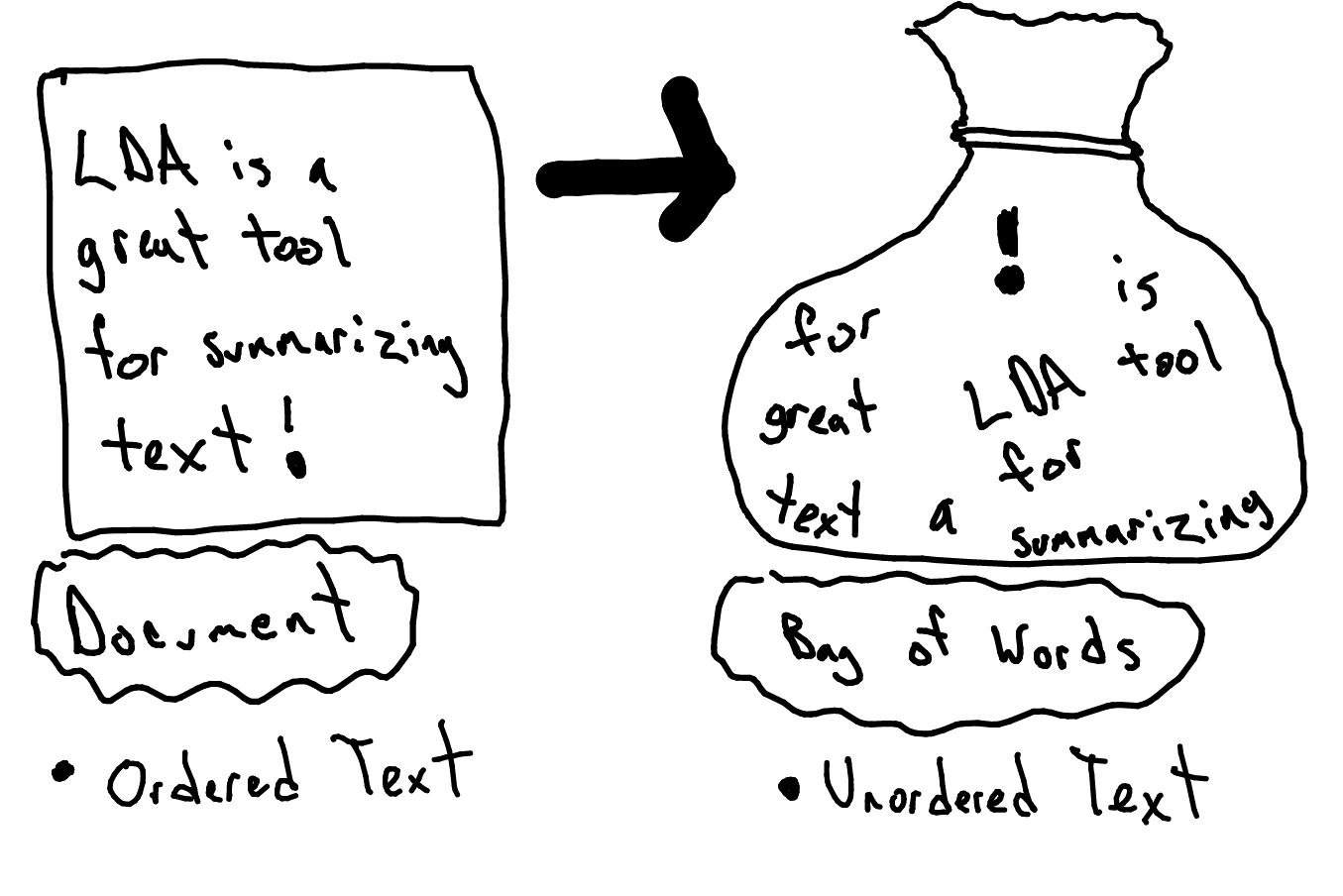# 中文

“我喜欢这个游戏”

“我 喜欢 这个 游戏”

I love the game.

I hate the game.

“我 喜欢 这个 游戏”

scikit-learn开发团队里，大概缺少足够多的中文使用者吧。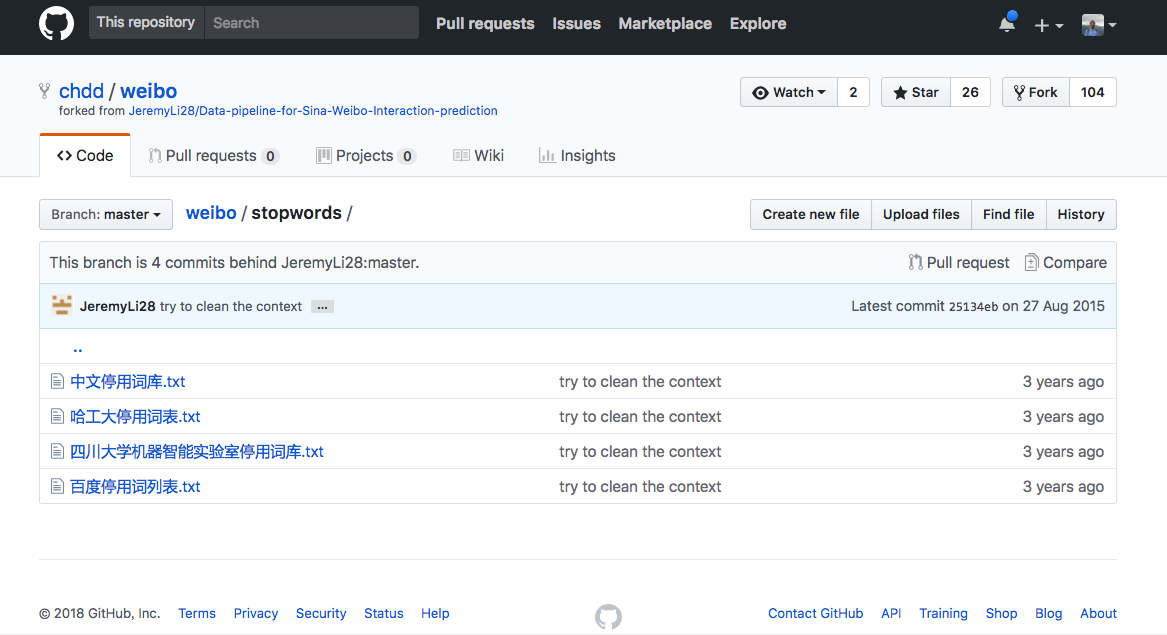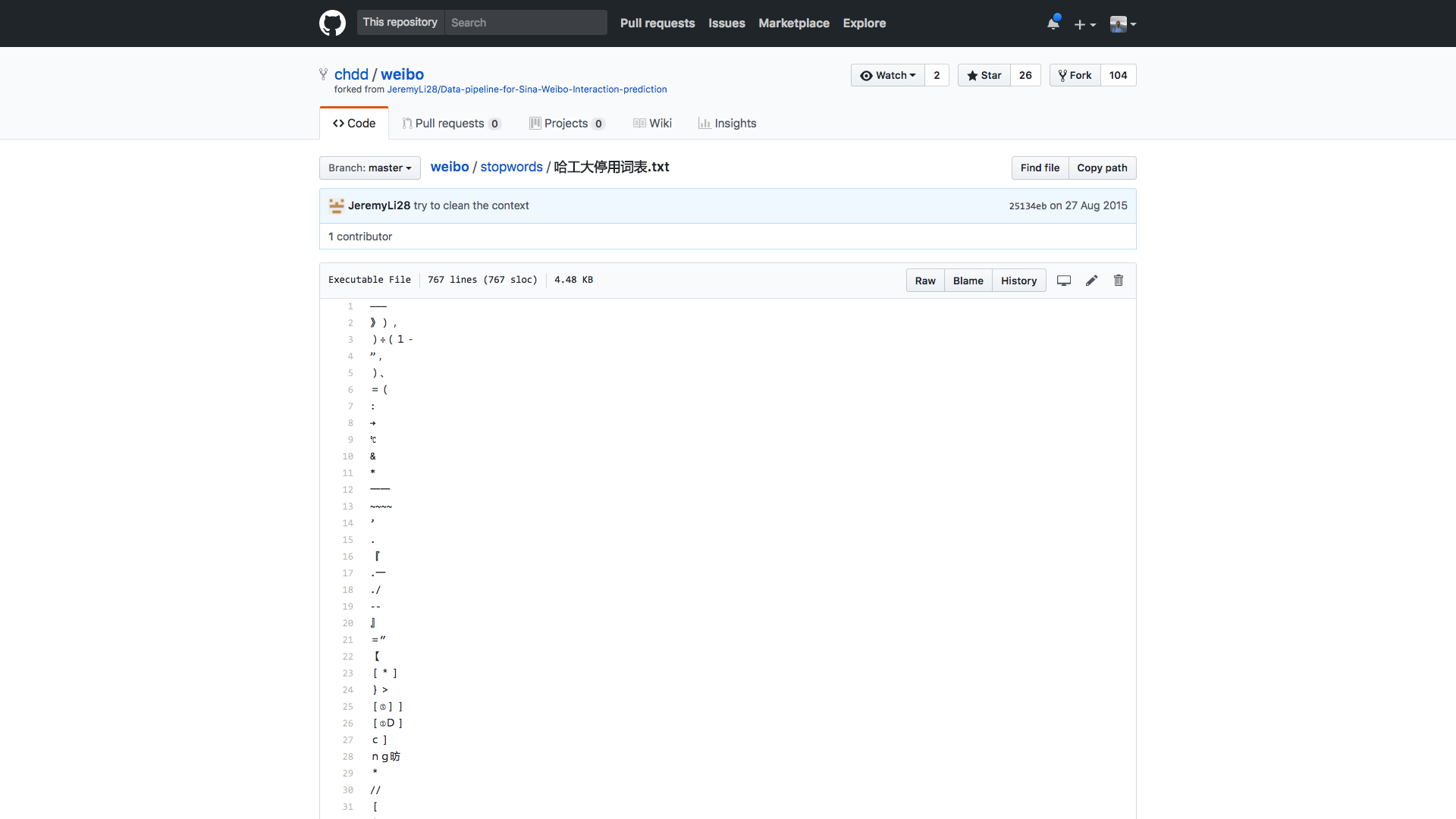# 环境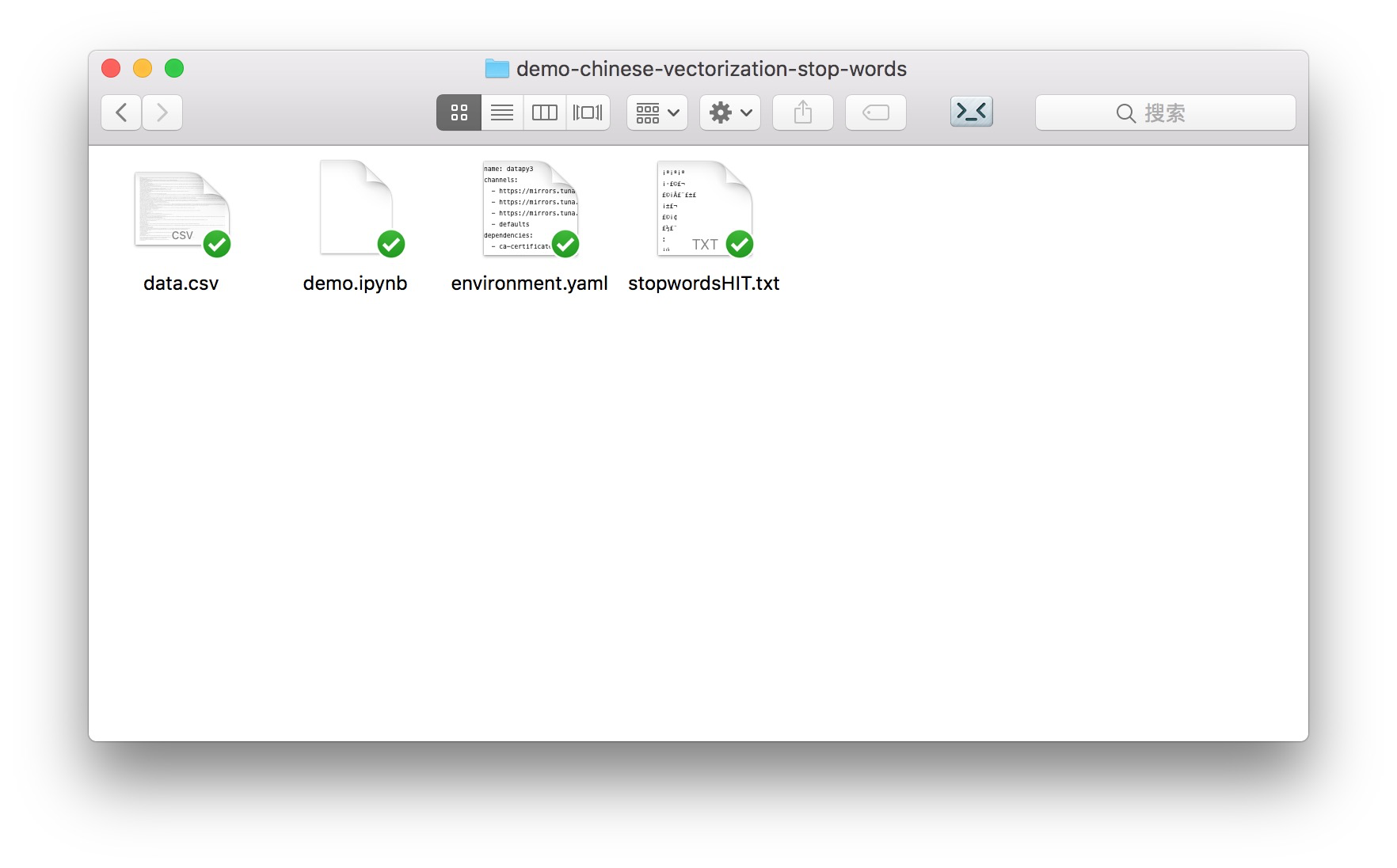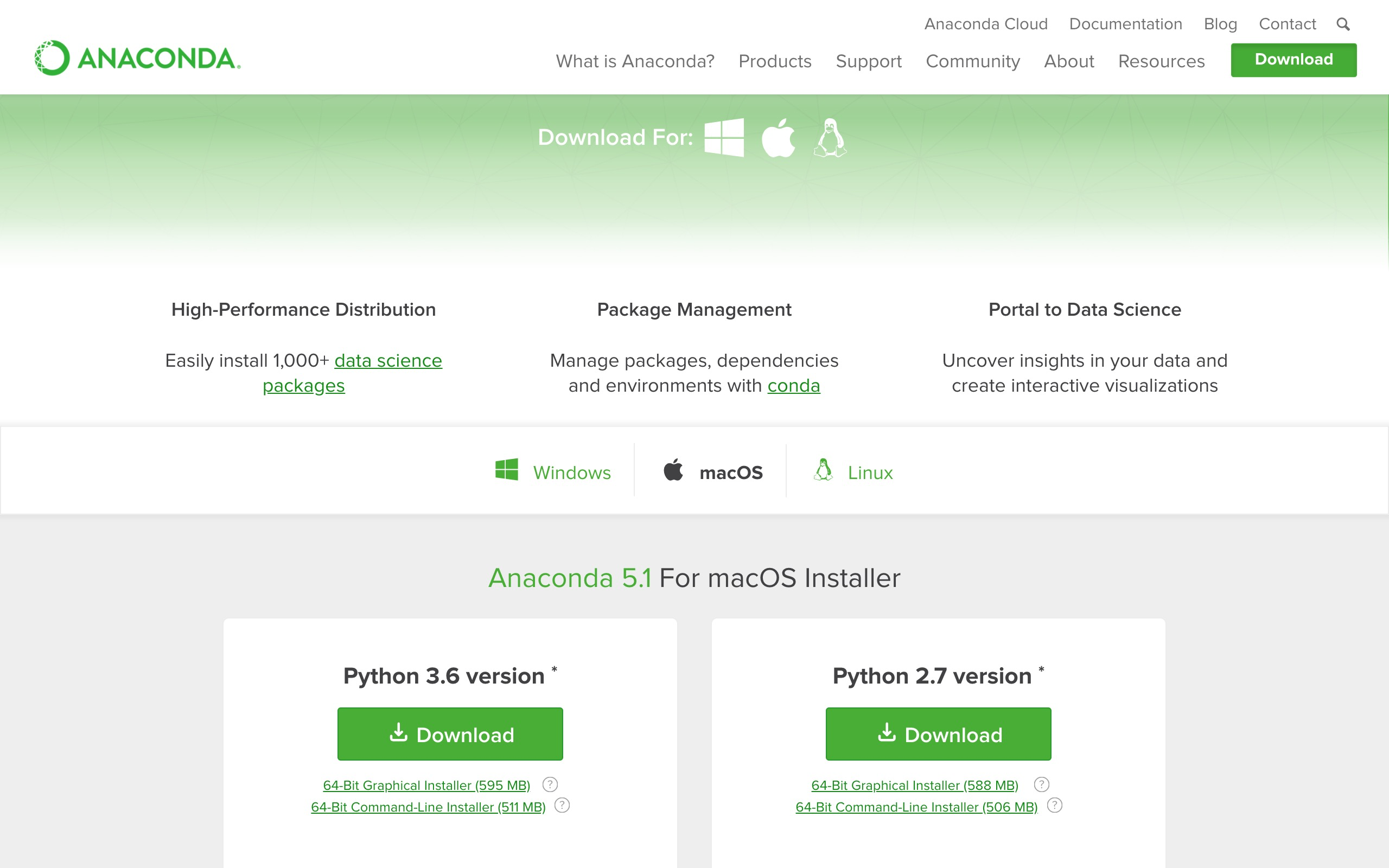conda env create -f environment.yaml

source activate datapy3

python -m ipykernel install --user --name=datapy3

jupyter notebook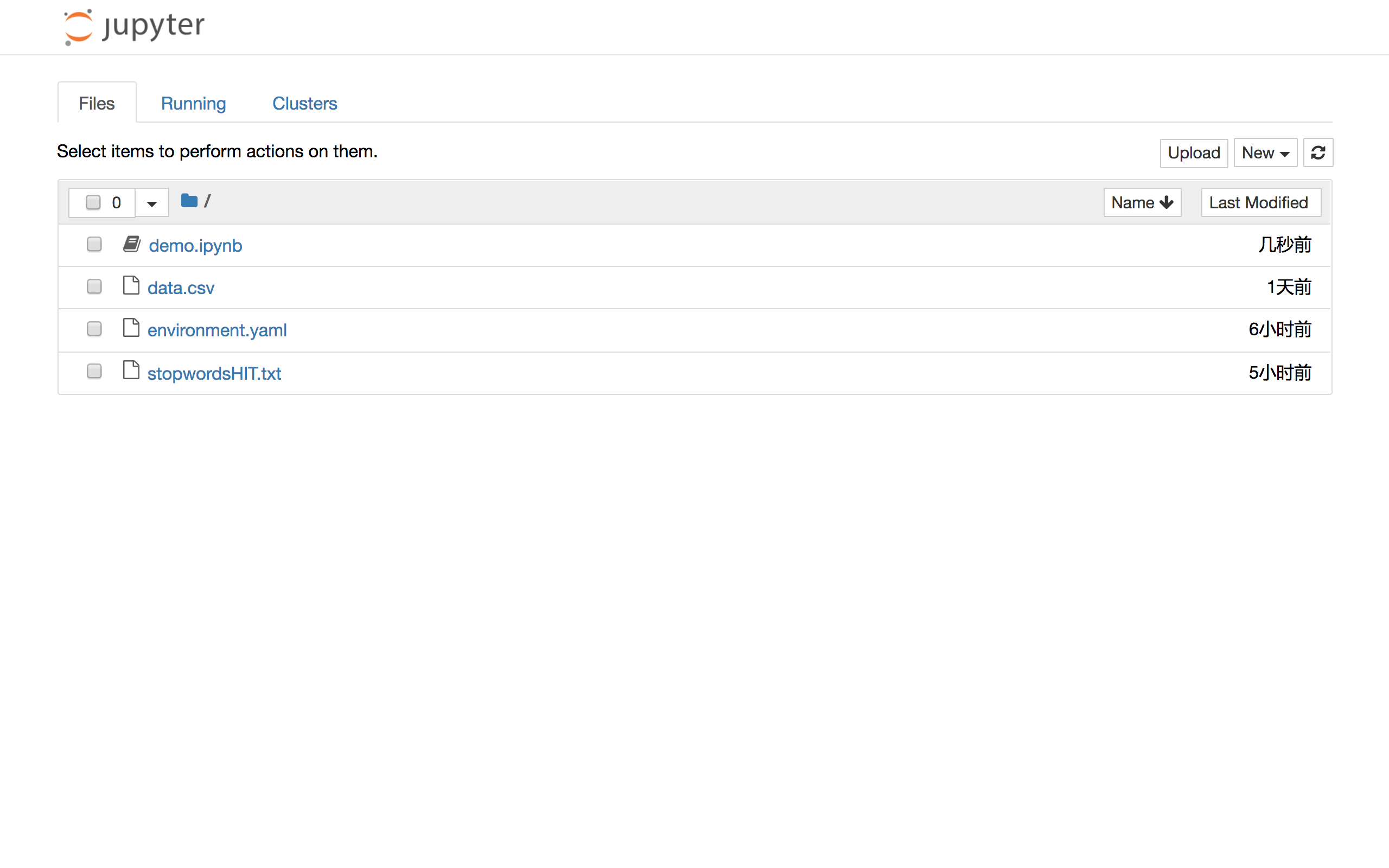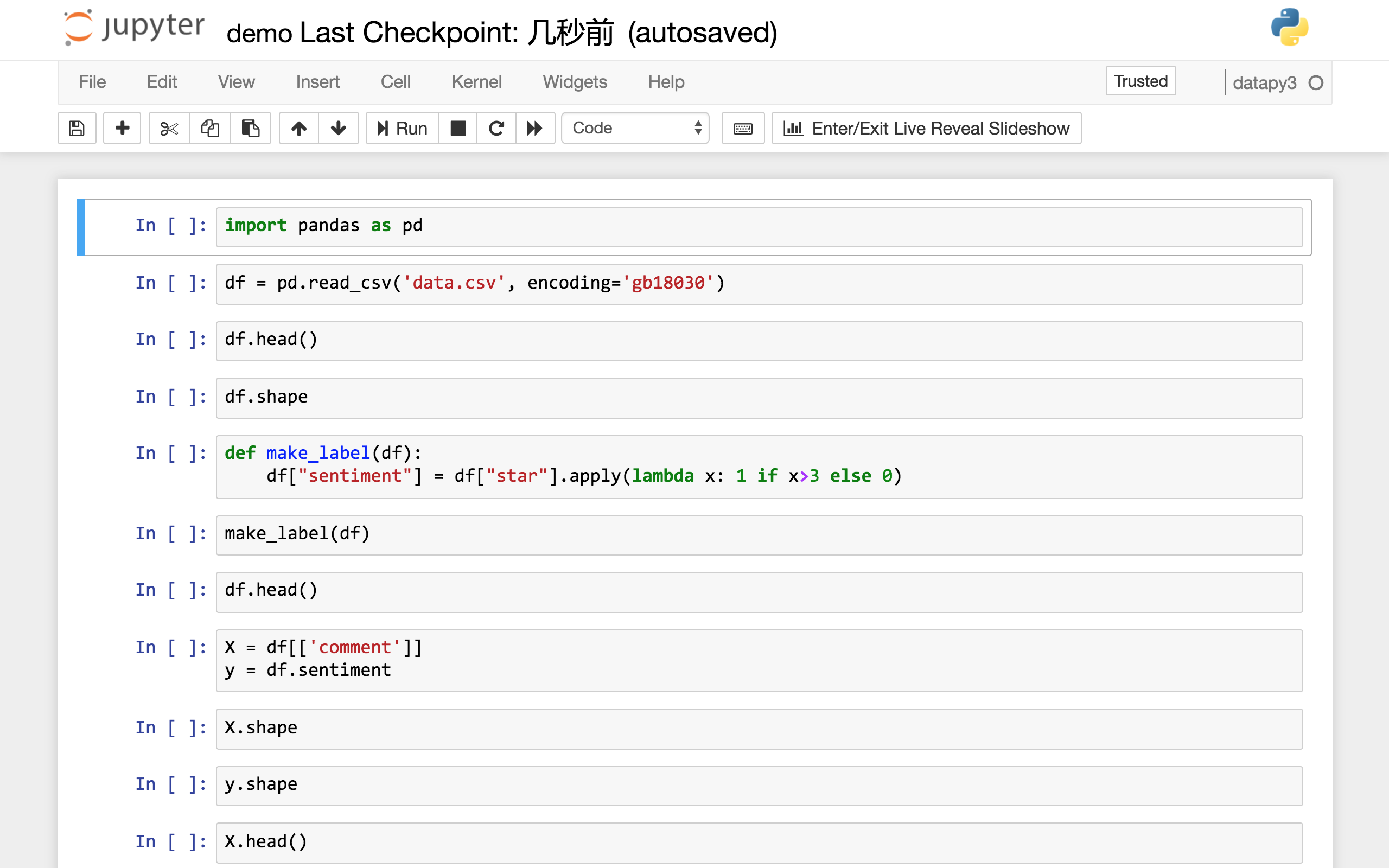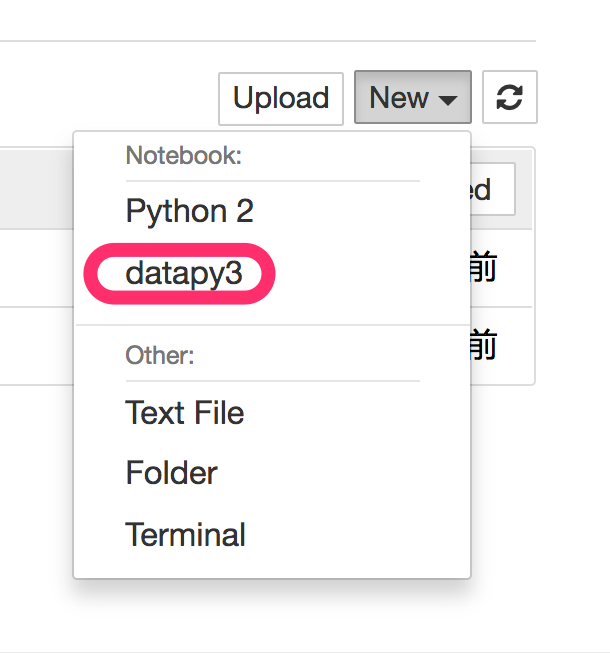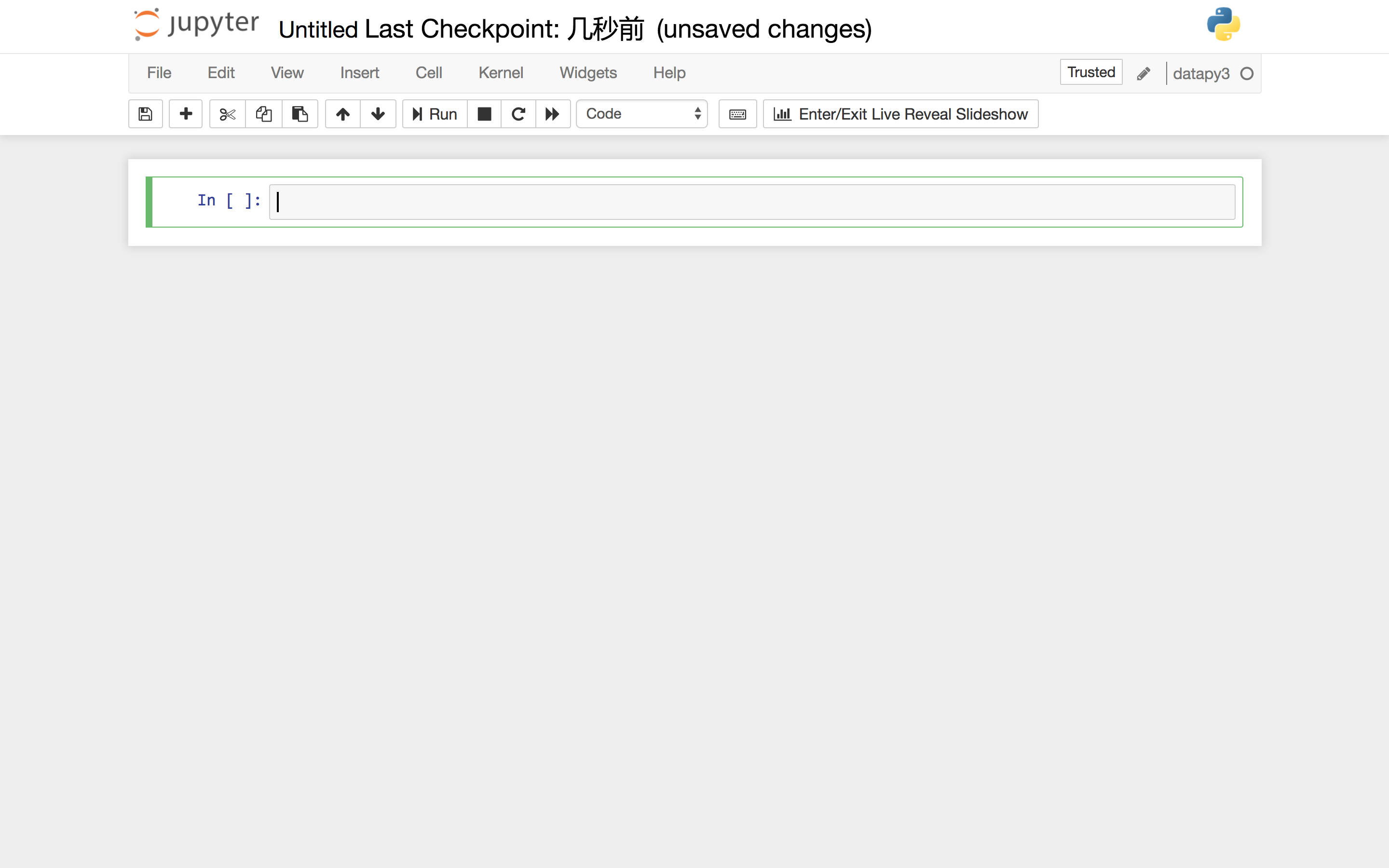# 代码

import pandas as pd


df = pd.read_csv('data.csv', encoding='gb18030')


df.head()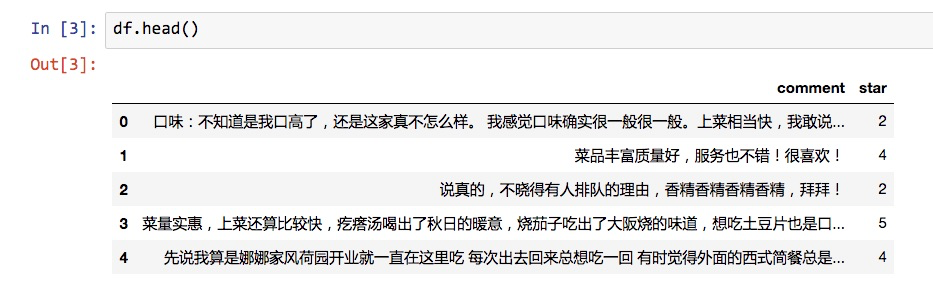df.shape

(2000, 2)


def make_label(df):
df["sentiment"] = df["star"].apply(lambda x: 1 if x>3 else 0)


make_label(df)


df.head()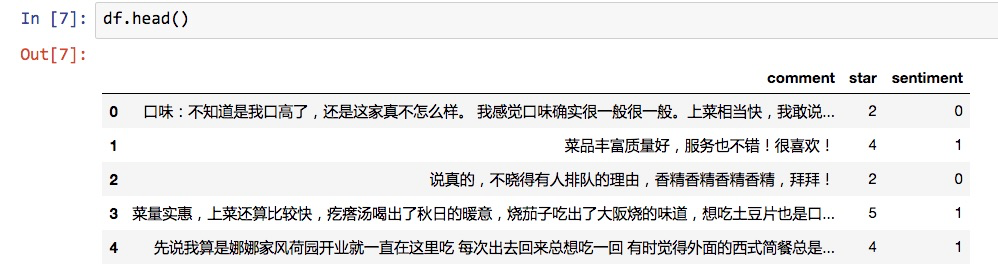X = df[['comment']]
y = df.sentiment


X 是我们的全部特征。因为我们只用文本判断情感，所以X实际上只有1列。

X.shape

(2000, 1)


y.shape

(2000,)


X.head()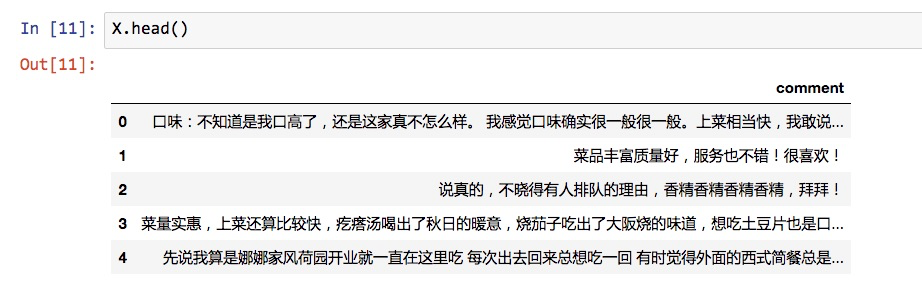import jieba


def chinese_word_cut(mytext):
return " ".join(jieba.cut(mytext))


X['cutted_comment'] = X.comment.apply(chinese_word_cut)


X.cutted_comment[:5]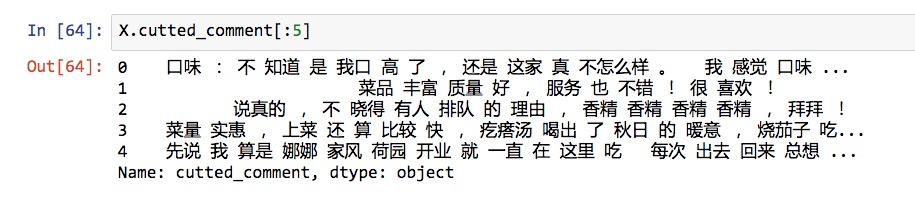from sklearn.model_selection import train_test_split
X_train, X_test, y_train, y_test = train_test_split(X, y, random_state=1)


X_train.shape

(1500, 2)


y_train.shape

(1500,)

X_test.shape

(500, 2)

y_test.shape

(500,)


def get_custom_stopwords(stop_words_file):
with open(stop_words_file) as f:
stopwords_list = stopwords.split('\n')
custom_stopwords_list = [i for i in stopwords_list]
return custom_stopwords_list


stop_words_file = "stopwordsHIT.txt"
stopwords = get_custom_stopwords(stop_words_file)


stopwords[-10:]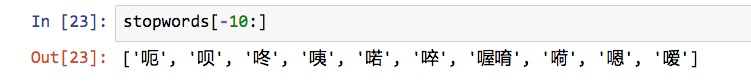from sklearn.feature_extraction.text import CountVectorizer


vect = CountVectorizer()


term_matrix = pd.DataFrame(vect.fit_transform(X_train.cutted_comment).toarray(), columns=vect.get_feature_names())


term_matrix.head()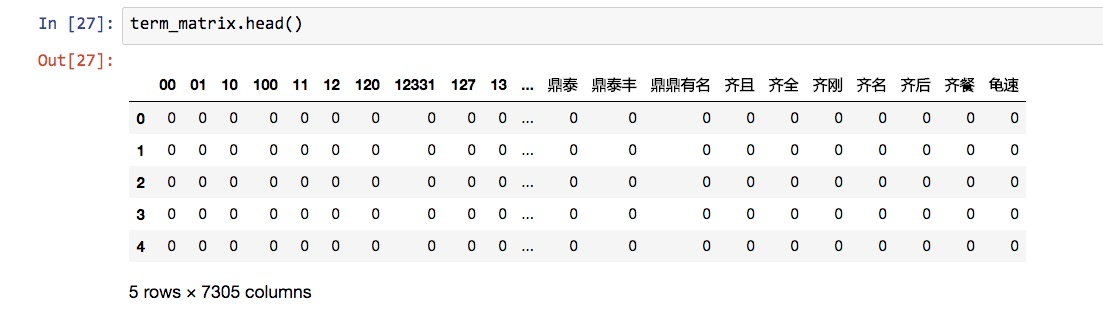term_matrix的形状如下：

term_matrix.shape

(1500, 7305)


vect = CountVectorizer(stop_words=frozenset(stopwords))


term_matrix = pd.DataFrame(vect.fit_transform(X_train.cutted_comment).toarray(), columns=vect.get_feature_names())

term_matrix.head()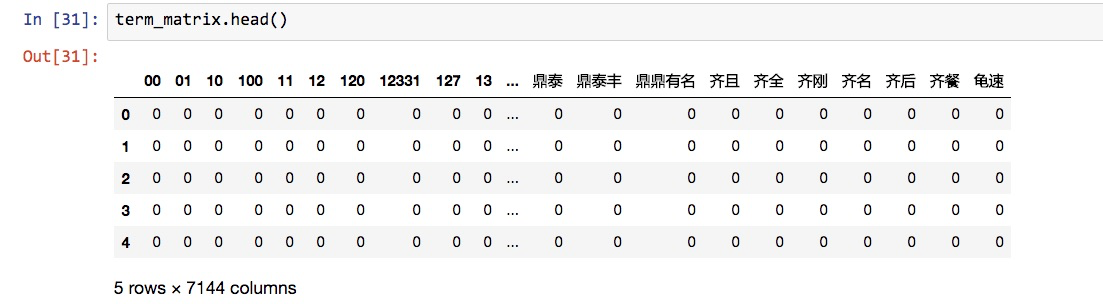max_df = 0.8 # 在超过这一比例的文档中出现的关键词（过于平凡），去除掉。
min_df = 3 # 在低于这一数量的文档中出现的关键词（过于独特），去除掉。

vect = CountVectorizer(max_df = max_df,
min_df = min_df,
token_pattern=u'(?u)\\b[^\\d\\W]\\w+\\b',
stop_words=frozenset(stopwords))


term_matrix = pd.DataFrame(vect.fit_transform(X_train.cutted_comment).toarray(), columns=vect.get_feature_names())

term_matrix.head()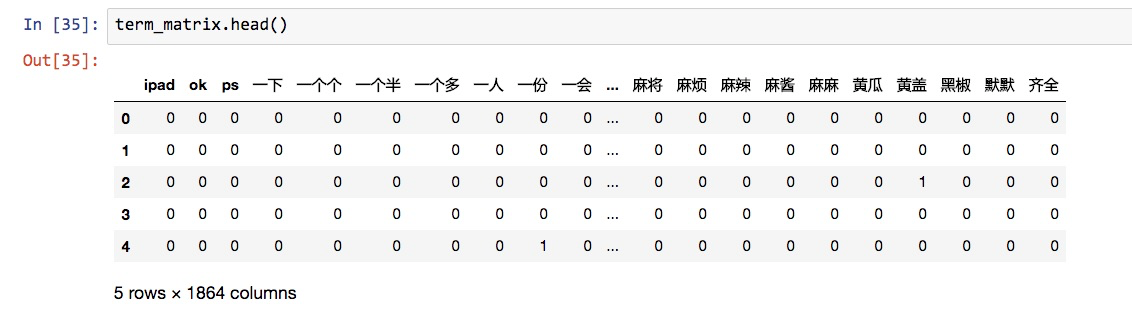from sklearn.naive_bayes import MultinomialNB
nb = MultinomialNB()


1. 特征向量化；
2. 朴素贝叶斯分类。

from sklearn.pipeline import make_pipeline
pipe = make_pipeline(vect, nb)


pipe.steps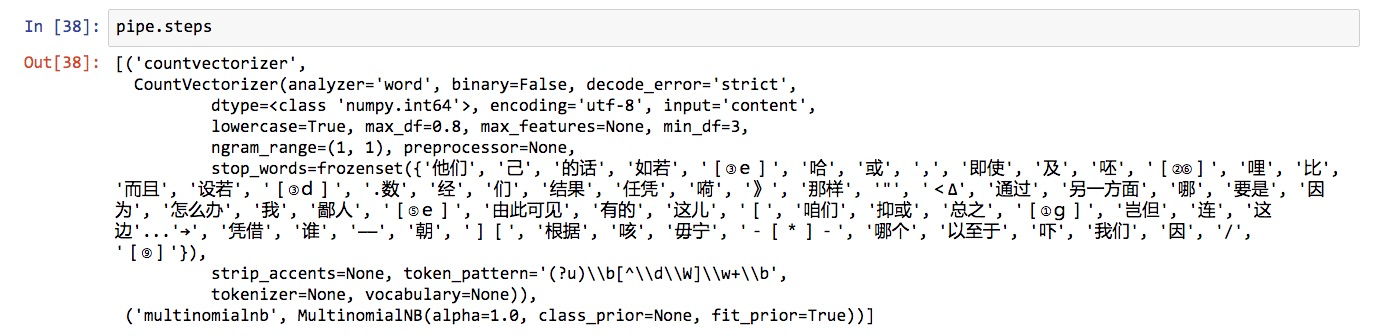from sklearn.cross_validation import cross_val_score
cross_val_score(pipe, X_train.cutted_comment, y_train, cv=5, scoring='accuracy').mean()


0.820687244673089


pipe.fit(X_train.cutted_comment, y_train)


pipe.predict(X_test.cutted_comment)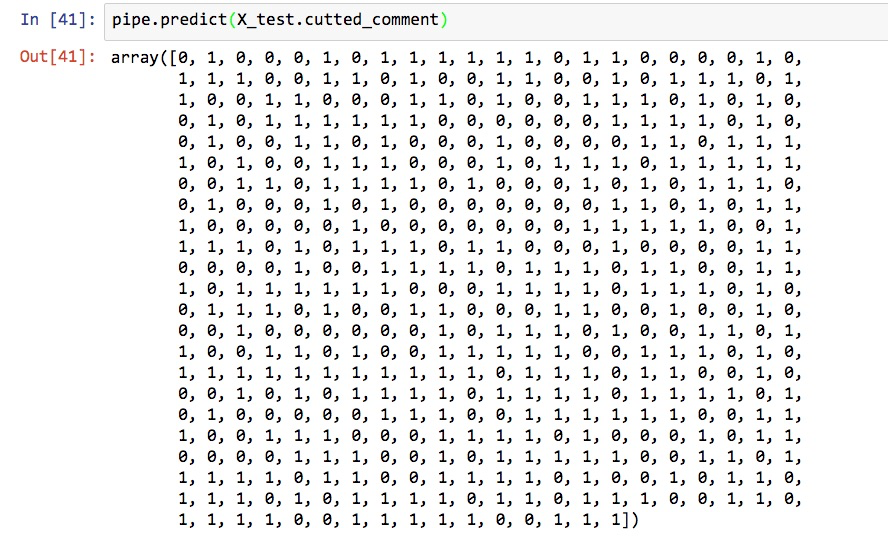y_pred = pipe.predict(X_test.cutted_comment)


from sklearn import metrics


metrics.accuracy_score(y_test, y_pred)

0.86


metrics.confusion_matrix(y_test, y_pred)

array([[194,  43],
[ 27, 236]])


• TP: 本来是正向，预测也是正向的；
• FP: 本来是负向，预测却是正向的；
• FN: 本来是正向，预测却是负向的；
• TN: 本来是负向，预测也是负向的。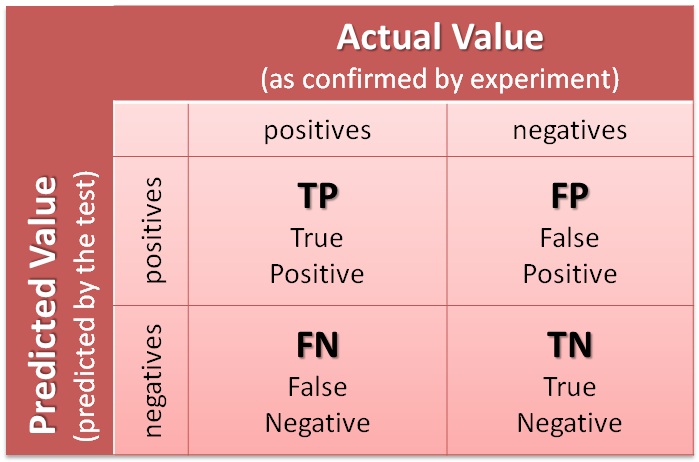from snownlp import SnowNLP
def get_sentiment(text):
return SnowNLP(text).sentiments


y_pred_snownlp = X_test.comment.apply(get_sentiment)


y_pred_snownlp_normalized = y_pred_snownlp.apply(lambda x: 1 if x>0.5 else 0)


y_pred_snownlp_normalized[:5]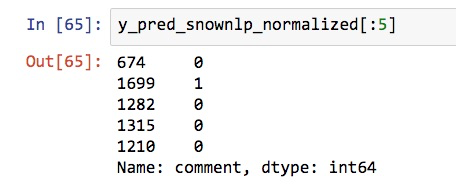metrics.accuracy_score(y_test, y_pred_snownlp_normalized)

0.77


metrics.confusion_matrix(y_test, y_pred_snownlp_normalized)

array([[189,  48],
[ 67, 196]])


# 小结

1. 如何用一袋子词（bag of words）模型将自然语言语句向量化，形成特征矩阵；
2. 如何利用停用词表、词频阈值和标记模式(token pattern)移除不想干的伪特征词汇，降低模型复杂度。
3. 如何选用合适的机器学习分类模型，对词语特征矩阵做出分类；
4. 如何用管道模式，归并和简化机器学习步骤流程；
5. 如何选择合适的性能测度工具，对模型的效能进行评估和对比。

# 讨论|
5天前
|

Python如何输出文本到屏幕
Python如何输出文本到屏幕
8 0
|
5天前
|
Python

10 0
|
1月前
|

Python读取Word/PPT文件文本内容和图片内容

54 0
|
1月前
|

2021-4月Python 机器学习——中文新闻文本标题分类
2021-4月Python 机器学习——中文新闻文本标题分类
40 0
|
1月前
|

2021-4月Python 机器学习——中文新闻文本标题分类(简单容易版)
2021-4月Python 机器学习——中文新闻文本标题分类(简单容易版)
48 0
|
2月前
|

46 0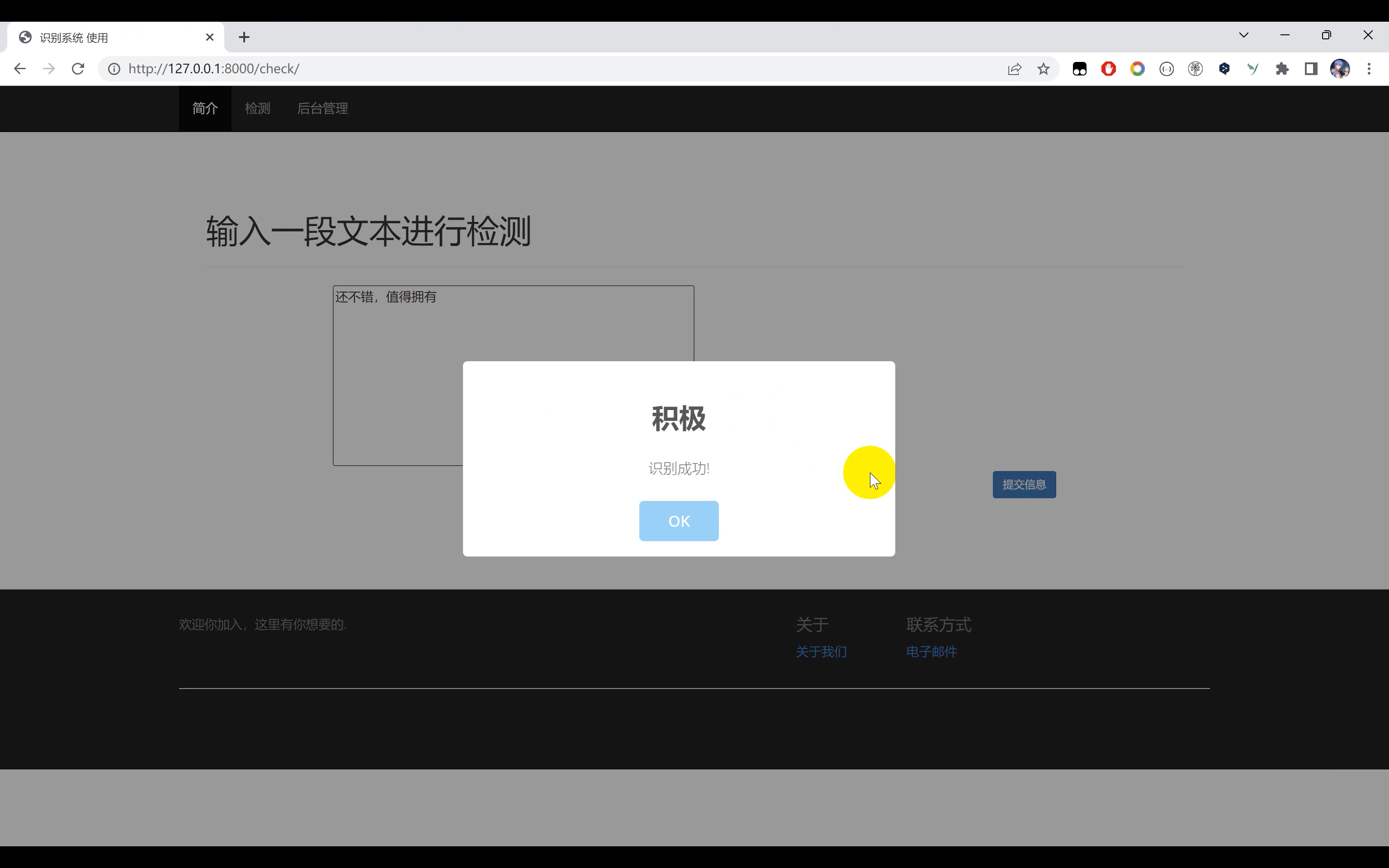|
3月前
|
Python
Python——获取网页文本内容
Python——获取网页文本内容
80 0
|
3月前
|
Python
Python统计文本词汇出现次数
Python统计文本词汇出现次数
85 0
|
3月前
|
API UED Python

91 0
|
4月前
|
JSON 关系型数据库 MySQL
【Python】【MySQL】Python将JSON数据以文本形式存放到MySQL的Text类型字段中
【Python】【MySQL】Python将JSON数据以文本形式存放到MySQL的Text类型字段中
104 0